Get instant live expert help with Excel or Google Sheets“My Excelchat expert helped me in less than 20 minutes, saving me what would have been 5 hours of work!”

#### Post your problem and you'll get expert help in seconds

Your message must be at least 40 characters
Our professional experts are available now. Your privacy is guaranteed.

# Fixed Value Every N Months

Excel allows a user to write a fixed value every n months in a defined column using several functions: IF, MOD and DATEDIF. This step by step tutorial will assist all levels of Excel users to assign a certain value every n months from a defined starting date.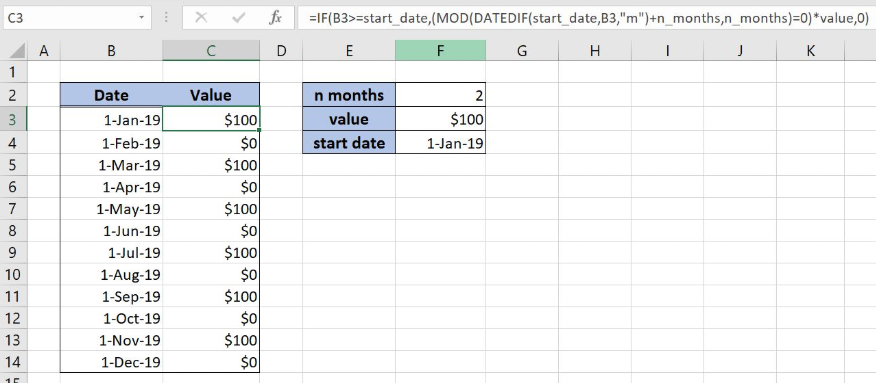Figure 1. Get fixed value every n months

## Syntax of the IF Formula

`=IF(logical_test, value_if_true, value_if_false)`

The parameters of the IF function are:

• logical_test – a logical expression that we want to check
• value_if_true – a value which the function returns if a logical_test is TRUE
• value_if_false – a value which the function returns if a logical_test is FALSE.

## Syntax of the MOD Formula

`=MOD(number,divisor)`

The parameter of the MOD function is:

• number – a number for which we want to find a remainder after the division
• divisor – a number that will be used as a divisor

## Syntax of the DATEDIF Formula

`=DATEDIF(start_date, end_date, unit)`

The parameters of the DATEDIF function are:

• start_date – a date from which we want to count the difference

• end_date -a date to which we want to count the difference
• unit – time unit in which we want to present the difference between the two dates (days, months, years)

## Setting up Our Data for the Formula

Let’s look at the structure of the data we will use. In column B (“Date”) we have dates, while in column C (“Values”) we will place the formula that assigns the fixed value every n months. In column F is data for the calculation. The “n months” is every other month for which the fixed value should be displayed.  The “value” is a \$100 value that will be displayed every other month while the “start date” is a date from which will be calculated every n month, 1-Jan-19.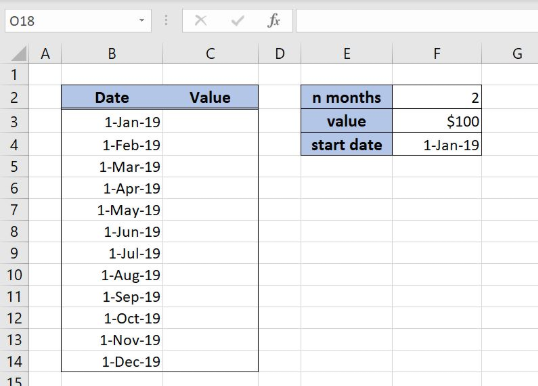Figure 2. Data that we will use in the example

## Assign the Fixed Value Every Other Month

The idea is to write \$100 in column C every other month starting from 1-Jan-19. For this purpose, we will use IF, DATEDIF and MOD functions. In order to make the formula more clear we will create three named ranges, n_months for the cell F2, value for the cell F3 and the start_date for the cell F4.

To create a named range we should follow the steps:

• Select the cell range that should be named
• Click on the name box in Excel
• Write the name for the cell range and press enter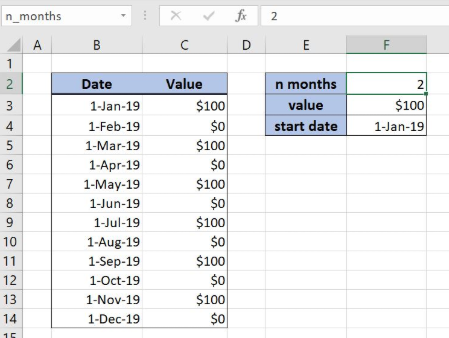Figure 3. Creating a named range n_months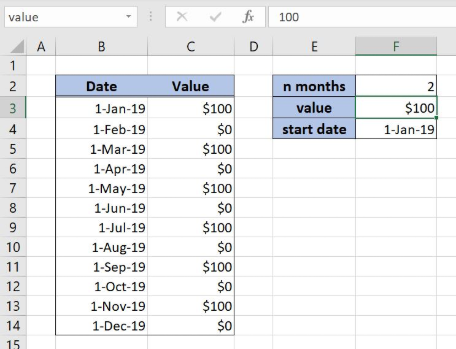Figure 4. Creating a named range value for the fixed value \$100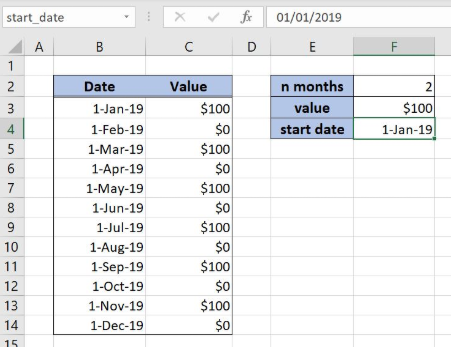Figure 5. Creating a named range start_date for the example

The formula looks like:

`=IF(B3>=start_date,(MOD(DATEDIF(start_date,B3,"m")+n_months,n_months)=0)*value,0)`

The logical_test in the IF function is checking if the date in the cell B3 is higher than the start_date, B3>=start_date. The parameter value_if_true is formula `(MOD(DATEDIF(start_date,B3,"m")+n_months,n_months)=0)*value`, while the value_if_false is 0.

In MOD function number is the formula `DATEDIF(start_date,B3,"m")+n_months`, while the parameter divisor is the named range n_months. In DATEDIF function strat_date is a named range start_date, while the end_date is the cell B3. The argument unit is an “m” since we want to get the difference in the months.

To apply the formula, we need to follow these steps:

• Select cell B3 and click on it
• Insert the formula: `=IF(B3>=start_date,(MOD(DATEDIF(start_date,B3,"m")+n_months,n_months)=0)*value,0)`
• Press enter
• Drag the formula down to the other cells in the column by clicking and dragging the little “+” icon at the bottom-right of the cell.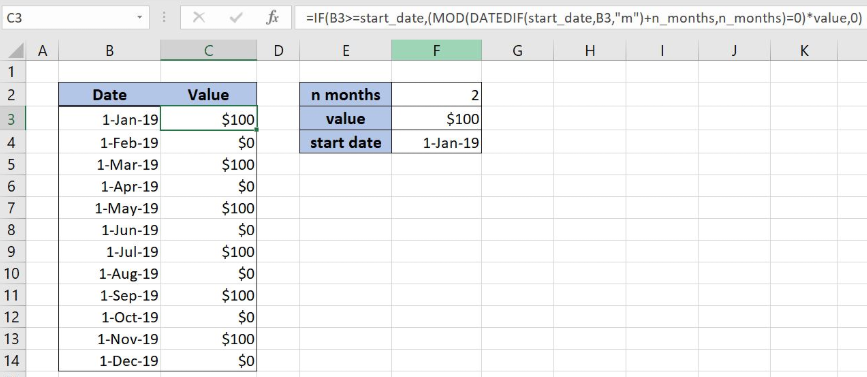Figure 6. Assign fixed value every other month with IF, MOD and DATEDIF functions

The DATEDIF function calculates the difference between the date in the cell B3 and the start date. The result is 0 months because the dates are the same. The MOD function gets the remainder of the DATEDIF function result increased by 2 and with the divisor 2. The output of the MOD formula is 0 because there is no remainder.

The IF function is checking if the date in the cell B3 is greater or equal to the start date. The condition is met and IF formula looks like IF(TRUE,(0=0)*value,0). Formula part 0=0 is checking if there is no a remainder in the MOD function. The result is TRUE and the formula looks like: `IF(TRUE,TRUE*100,0)`. The TRUE is interpreted as number 1 and formula output is \$100.

Most of the time, the problem you will need to solve will be more complex than a simple application of a formula or function. If you want to save hours of research and frustration, try our live Excelchat service! Our Excel Experts are available 24/7 to answer any Excel question you may have. We guarantee a connection within 30 seconds and a customized solution within 20 minutes.

### Did this post not answer your question? Get a solution from connecting with the expert.Another blog reader asked this question today on Excelchat:## Subscribe to Excelchat.coAnother blog reader asked this question today on Excelchat: